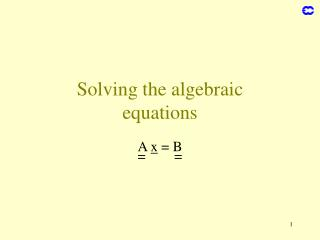# Solving the algebraic equations - PowerPoint PPT PresentationDownload PresentationSolving the algebraic equations

Solving the algebraic equations
Download Presentation## Solving the algebraic equations

- - - - - - - - - - - - - - - - - - - - - - - - - - - E N D - - - - - - - - - - - - - - - - - - - - - - - - - - -
##### Presentation Transcript

1. Solving the algebraicequations A x = B = =

2. Direct solution x = A-1 B = = • Applicable only to small problems • For the vertical in the spectral technique where x is a one-column vector (decoupled equations in the horizontal)

3. substitute in the 2nd equation extract x2 Gauss elimination Tridiagonal matrices: Large one-dimensional problems substitute in the 3rd equation …. and so on solve and substitute in the (n-1)th eq. we arrive at a single equation for xn solve for xn-1 and substitute in the (n-2)th eq. etc …….. Pivots: a11 , a22-a21/a11 , … not too small (might need to rearrange order)

4. - Correct from the value of is small enough - continue until The method converges if Iterative methods Guess a solution

5. pre-condition system add and substract * if is the true solution continuous equivalent of * the general solution of this equation is: where the λ’s are the eigenvalues of matrix General iterative procedure • Convergence it approaches the stationary solution k if Re(λ) < 0 (elliptic problem)

6. Example of iterative procedure Helmholtz equation in finite differences we have taken Δx=1 for simplicity then take * where means all x from iteration nexcept xi,j from iteration n+1 this is the Jacobi method if we take xi-1,j and xi,j-1 from iteration n+1, we have the Gauss-Seidel method multiplying the correction in * by a factor μ>1, we have the overrelaxation method

7. The overrelaxation method R x x x x j

8. Multigrid methods • An iterative scheme is slow if the corrections from the initial guess are long-range corrections but very fast if they are local • Multigrid methods first relax on a subset of the grid(therefore long-range corrections cover a lesser number of grid-points and are seen as more local)and then refine, relaxing on the original grid(or an intermediate one …) and the switching between grids is iterated • This procedure is much more efficient than the straightforward relaxation and can compete with direct methods • It is even more efficient in multiprocessor machines • Adaptive multigrid methods only refine in the areas where the error is larger than a given threshold

9. Multigrid methods (2) long-range errors and sampling short-range errors R R x x x x x

10. Decoupling the equations Assume we have a 3-D problem tensor product • Simplest case that is auxiliary vectors solve for each (m,n). Then solve for each (i,n). Finally solve for each (i,j). Total O(I.J.K)3 operations

11. Decoupling the equations (cont) • Use of the eigenvector matrix Consider the Poisson equation in 3 dimensions Using centered finite diff. In the vertical: where Is a matrix of rank K (No of levels)

12. Decoupling the equations (cont) Let be the eigenvectors of calling the matrix formed by the eigenvectors being the diagonal matrix of eigenvalues The discretized equation can then be written as: K decoupled equations projections of φ along the eigenvectors

13. Fourier transform method Consider the 2-dimensional Poisson equation in finite-differences or where here Un: grid-point values of U in row n

14. The same holds for any other matrix of the form (Helmholtz equation) Fourier transform method (cont) A is a tridiagonal symmetric matrix whose eigenvalues are j=1, 2, …, M and the eigenvectors are the Fourier basis functions

15. Fourier transform method (cont2) Call and The original system may be written as follows: Discrete Fourier transform of vector of grid-points at row k+1 decoupled system of equations for the Fourier components (k=row number) The projection to Fourier space and back can be done by FFT

16. Thank you Any question?